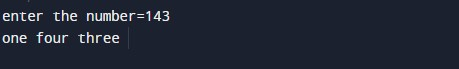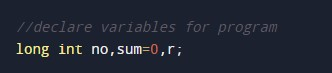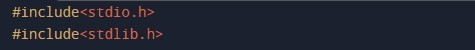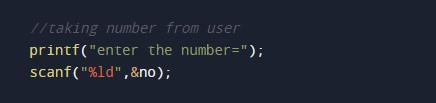# C Program to Convert Number into Word

In this tutorial you will learn about the C Program to Convert Number into Word and its application with practical example.

## C Program to Convert Number into Word

In this tutorial, we will learn to create a C program that will convert Number into Word  using C programming.

## Prerequisites

Before starting with this tutorial we assume that you are best aware of the following C programming topics:

• Operators in C Programming.
• Basic Input and Output function in C Programming.
• Basic C programming.
• While loop in c programming.
• Switch Case statement in C Programming.
• Header Libraries and its usage.

## Convert Number into Word

In c programming it is possible to take numerical input from the user and convert it into words  with the help of very small amount of code . The C language has many types of header libraries which has supported function in them with the help of these files the programming is easy.

With the help of this program we can Convert Number into Word.

## Output:-The above program we have first initialize the required variable.• no = it will hold the integer value.
• r = it will hold the value of digit.
• sum = it will hold the sum.

Including the header files required for program.Input number from user.Switch Case body.In this tutorial we have learn about the C Program to Convert Number into Word and its application with practical example. I hope you will like this tutorial.